# HOMER Pro 3.12

 Navigation: Glossary Hydro Turbine Flow RateType: Intermediate Variable Units: m3/s Symbol: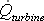The hydro turbine flow rate is the amount of water flowing through the hydro turbine. HOMER calculates this value in each time step using the following equation: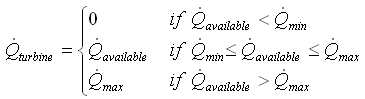where: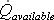[m3/s]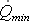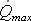Note: As this equation shows, HOMER assumes that unless the available stream exceeds the turbine's minimum flow rate, the turbine flow rate is zero, meaning the turbine does not operate and hence produces no power. If HOMER reports that your hydro turbine is not producing any power, this is probably the reason.

HOMER uses the hydro turbine flow rate to calculate the hydro power output in each time step.

See also

How HOMER Calculates the Hydro Power Output